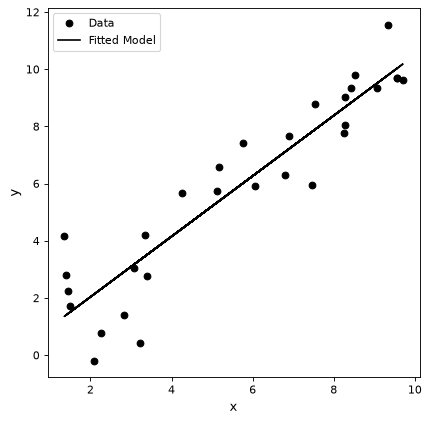# Models and Fitting (`astropy.modeling`)¶

## Introduction¶

`astropy.modeling` provides a framework for representing models and performing model evaluation and fitting. A number of predefined 1-D and 2-D models are provided and the capability for custom, user defined models is supported. Different fitting algorithms can be used with any model. For those fitters with the capabilities fitting can be done using uncertainties, parameters with bounds, and priors.

## A Simple Example¶

This simple example illustrates defining a model, calculating values based on input x values, and using fitting data with a model.

```import numpy as np
import matplotlib.pyplot as plt
from astropy.modeling import models, fitting

# define a model for a line
line_orig = models.Linear1D(slope=1.0, intercept=0.5)

# generate x, y data non-uniformly spaced in x
# add noise to y measurements
npts = 30
np.random.seed(10)
x = np.random.uniform(0.0, 10.0, npts)
y = line_orig(x)
y += np.random.normal(0.0, 1.5, npts)

# initialize a linear fitter
fit = fitting.LinearLSQFitter()

# initialize a linear model
line_init = models.Linear1D()

# fit the data with the fitter
fitted_line = fit(line_init, x, y)

# plot the model
plt.figure()
plt.plot(x, y, 'ko', label='Data')
plt.plot(x, fitted_line(x), 'k-', label='Fitted Model')
plt.xlabel('x')
plt.ylabel('y')
plt.legend()
```

(png, svg, pdf)## Pre-Defined Models¶

Some of the pre-defined models are listed and illustrated.